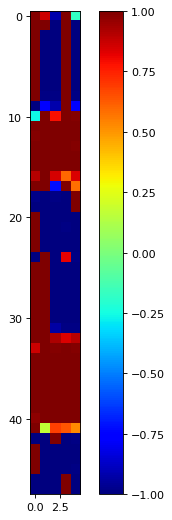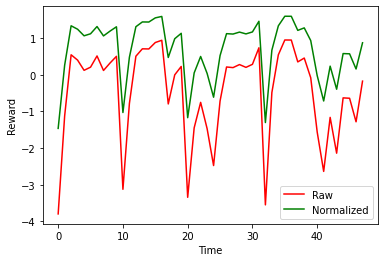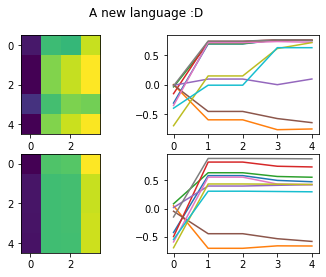# CommNet and DDPG

Implementing DDPG and CommNet

A notebook containing a sample starter implementation of a centralised agent utilising the DDPG algorithm for training.

This is not interfaced with orderenforcingwrapper.py.

# Initialization¶

Related imports and setup.

This notebook demonstrates how to use a basic multiagent reinforcement learning algorithm to tackle the CityLearn 2022 problem, with an implementation of CommNet and centralised DeepDeterministicPolicyGradient. I assume basic reader familiarity with RL concepts.

CommNet: S Sukhbaata, Learning Multiagent Communication with Backpropagation, https://arxiv.org/abs/1605.07736

DDPG: TP Lillicrap, Continuous Control with Deep Reinforcement Learning, https://arxiv.org/abs/1509.02971

In :
import numpy as np
import math
import time, random, typing, cProfile, traceback
import matplotlib.pyplot as plt
import torch
import torch.nn as nn
import torch.nn.functional as F
import torch.optim as optim
import copy
import pandas as pd

from citylearn.citylearn import CityLearnEnv

class Constants:
schema_path = './data/citylearn_challenge_2022_phase_1/schema.json'

def action_space_to_dict(aspace):
""" Only for box space """
return { "high": aspace.high,
"low": aspace.low,
"shape": aspace.shape,
"dtype": str(aspace.dtype)
}

def env_reset(env):
observations = env.reset()
action_space = env.action_space
observation_space = env.observation_space
building_info = env.get_building_information()
building_info = list(building_info.values())
action_space_dicts = [action_space_to_dict(asp) for asp in action_space]
observation_space_dicts = [action_space_to_dict(osp) for osp in observation_space]
obs_dict = {"action_space": action_space_dicts,
"observation_space": observation_space_dicts,
"building_info": building_info,
"observation": observations }
return obs_dict

env = CityLearnEnv(schema=Constants.schema_path)
obs_dict = env_reset(env)

device = torch.device("cuda" if torch.cuda.is_available() else "cpu")


# Algorithm¶

A very high level and not very rigorous overview.

### DDPG¶

A reinforcement learning algorithm combining insights from 3 seperate ideas.

I learnt a lot during implementation from Chris Yoon's article - https://towardsdatascience.com/deep-deterministic-policy-gradients-explained-2d94655a9b7b

#### Actor Critic Framework¶

A reinforcement learning framework using two seperate networks, answering two different questions.

1. Critic: How good is taking an action $A$ in state $S$? This network is trained by previous observations of the environment.
2. Actor: Given the state of the current environment, what is the best action to take? We can train this with the critic acting as a guide.

A special case of the policy gradient concerning a deterministic policy, which is a policy that outputs a single action rather than a distribution. This (counterintuitively) is a method that helps generalize DQN to continuous action spaces, and also allows us to utilize the gradient of the critic network during policy training.

#### Deep Q Networks¶

Given a perfect critic $Q$, the best action would be the argmax of the critic. Deep Q networks uses a neural network to approximate the critic on continuous state spaces, improving training with techniques such as a replay buffer.

DDPG extends deep Q networks to a continuous action space by introducing the actor-critic framework and DPG. Some key ideas...

1. DQN (and thus DDPG) is inherently off-policy, so it is possible to introduce a replay buffer to improving sample efficiency.
2. Target networks to improve training stability.

### CommNet¶

CommNet a deep learning architecture that offers a method for decentralised agents to learn a communication strategy. Actual execution of the policy looks more like a centralised agent (DDPG is easier to implement :D), but it is computationally efficient wrt. the number of agents and something I wanted to try out as a baseline.

1. Each agent computes a hidden state from local observation.
2. For N communication rounds... 2.1 Each agent uses its hidden state to calculate a communication vector. 2.2 The mean of all vectors (excluding the agent itself) is recieved to decide on a new hidden state.
3. We can then go with a policy from the hidden state to the action space.
In :
# Local directory structure - I haven't tested directly running this notebook yet.
'''
from agents.network.comm_net import CommNet
from agents.network.critic import SingleCritic
from util.normalizer import MinMaxNormalizer
'''

class CommNet(nn.Module):
'''
Implements CommNet for a single building
Of the CityLearn challenge
LSTM version with skip connection for the final layer

TODO: Try basic version without LSTM / alter skip connections etc
But might be a better idea to explore more advanced architectures instead
'''

def __init__(
self,
agent_number,       # Number of buildings present
input_size,         # Observation accessible to each building (assuming homogenous)
hidden_size = 10,   # Hidden vector accessible at each communication step
comm_size = 4,      # Number of communication channels
comm_steps = 2      # Number of communication steps
):

super(CommNet, self).__init__()

self.device = 'cpu'
self.input_size = input_size
self.comm_size = comm_size
self.agent_number = agent_number
self.comm_steps = comm_steps

# Calculate first hidden layer
self._in_mlp = nn.Sequential(
nn.Linear(input_size,input_size),
nn.LeakyReLU(),
nn.BatchNorm1d(input_size),
nn.Linear(input_size,input_size),
nn.LeakyReLU(),
nn.BatchNorm1d(input_size),
nn.Linear(input_size,hidden_size)
)

# Communication
self._lstm = nn.LSTMCell(
input_size = comm_size,
hidden_size = hidden_size
)

self._comm_mlp = nn.Sequential(
nn.Linear(hidden_size,hidden_size),
nn.LeakyReLU(),
nn.Linear(hidden_size,comm_size)
)

# Output
# Calculate based on inputs and final memory
self._out_mlp = nn.Sequential(
nn.Linear(input_size+hidden_size, input_size+hidden_size),
nn.LeakyReLU(),
nn.Linear(input_size+hidden_size, input_size+hidden_size),
nn.LeakyReLU(),
nn.Linear(input_size+hidden_size, 1),
nn.Tanh()
)

def forward(self,x : torch.Tensor, batch = False):

out = None
if not batch:

# (Building, Observations)

# Initial hidden states
hidden_states = self._in_mlp(x)
cell_states = torch.zeros(hidden_states.shape,device=self.device)

# Communication
for t in range(self.comm_steps):
# Calculate communication vectors
comm = self._comm_mlp(hidden_states)
total_comm = torch.sum(comm,0)
comm = (total_comm - comm) / (self.agent_number-1)
# Apply LSTM
hidden_states, cell_states = self._lstm(comm,(hidden_states,cell_states))

out = self._out_mlp(torch.cat((x,hidden_states),dim=1))
else:
# (Batch, Building, Observation)
out = torch.stack([self.forward(a) for a in x])

return out

def to(self,device):
super().to(device)
self.device = device

class SingleCritic(nn.Module):

def __init__(self,
input_size,
action_size = 1,
hidden_layer_size = 32):
super(SingleCritic, self).__init__()

self.input_size = input_size
self.action_size = action_size

self._in_mlp = nn.Sequential(
nn.Linear(input_size + action_size, hidden_layer_size),
nn.LeakyReLU(),
nn.Linear(hidden_layer_size, hidden_layer_size),
nn.LeakyReLU(),
nn.Linear(hidden_layer_size, 1),
)

def forward (self, state, action):
x = torch.cat((torch.flatten(state,start_dim=1),torch.flatten(action,start_dim=1)),dim=1)
return self._in_mlp(x)

from sklearn.preprocessing import MinMaxScaler

class MinMaxNormalizer:

def __init__(self, obs_dict):
observation_space = obs_dict['observation_space']
low, high = observation_space['low'],observation_space['high']

self.scalar = MinMaxScaler()
self.scalar.fit([low,high])

def transform(self, x):
return self.scalar.transform(x)

# Experience replay needs a memory - this is it!
# Double stack implementation of a queue - https://stackoverflow.com/questions/69192/how-to-implement-a-queue-using-two-stacks
class Queue:
a = []
b = []

def enqueue(self, x):
self.a.append(x)

def dequeue(self):
if len(self.b) == 0:
while len(self.a) > 0:
self.b.append(self.a.pop())
if len(self.b):
return self.b.pop()

def __len__(self):
return len(self.a) + len(self.b)

def __getitem__(self, i):
if i >= self.__len__():
raise IndexError
if i < len(self.b):
return self.b[-i-1]
else:
return self.a[i-len(self.b)]

class DDPG:
MEMORY_SIZE = 10000
BATCH_SIZE = 128
GAMMA = 0.95
LR = 3e-4
TAU = 0.001

memory = Queue()

def to(self,device):
self.device = device
self.actor.to(device)
self.actor_target.to(device)
self.critic.to(device)
self.critic_target.to(device)

def __init__(self, obs_dict):

N = len(obs_dict['building_info'])
obs_len = len(obs_dict['observation_space']['high'])

# Initalize actor networks
self.actor = CommNet(
agent_number=N,
input_size=obs_len
)

self.actor_target = copy.deepcopy(self.actor)

# Initialize critic networks
self.critic = SingleCritic(
input_size=obs_len*N,
action_size=N
)

self.critic_target = copy.deepcopy(self.critic)

self.normalizer = MinMaxNormalizer(obs_dict=obs_dict)

self.c_criterion = nn.MSELoss()

self.to("cpu")

def compute_action(self, obs, exploration=True, exploration_factor = 0.3):
obs = self.normalizer.transform(obs)
action = self.actor(torch.tensor(obs,device=self.device).float()).detach().cpu().numpy()
if exploration:
action = action + np.random.normal(scale=exploration_factor,size = action.shape)
action = np.clip(action,a_min=-1.0,a_max=1.0)
return action

def add_memory(self, s, a, r, ns):
s = self.normalizer.transform(s)
ns = self.normalizer.transform(ns)
self.memory.enqueue([s,a,r,ns])
if len(self.memory) > self.MEMORY_SIZE:
self.memory.dequeue()

def clear_memory(self):
self.memory.a = []
self.memory.b = []

# Conduct an update step to the policy
def update(self):

N = self.BATCH_SIZE
if len(self.memory) < 1: # Watch before learn
return
# Get a minibatch of experiences
# mb = random.sample(self.memory, min(len(self.memory),N)) # This is slow with a large memory size
mb = []
for _ in range(min(len(self.memory),N)):
mb.append(self.memory[random.randint(0,len(self.memory)-1)])

s = torch.tensor(np.array([x for x in mb]),device=self.device).float()
a = torch.tensor(np.array([x for x in mb]),device=self.device).float()
r = torch.tensor(np.array([x for x in mb]),device=self.device).float()
ns = torch.tensor(np.array([x for x in mb]),device=self.device).float()

# Critic update
nsa = self.actor_target.forward(ns,batch=True)
y_t = torch.add(torch.unsqueeze(r,1), self.GAMMA * self.critic_target(ns,nsa))
y_c = self.critic(s,a)
c_loss = self.c_criterion(y_c,y_t)
critic_loss = c_loss.item()
c_loss.backward()
self.c_optimize.step()

# Actor update
a_loss = -self.critic(s,self.actor.forward(s,batch=True)).mean() # Maximize gradient direction increasing objective function
a_loss.backward()
self.a_optimize.step()

# Target networks
for ct_p, c_p in zip(self.critic_target.parameters(), self.critic.parameters()):
ct_p.data = ct_p.data * (1.0-self.TAU) + c_p.data * self.TAU

for at_p, a_p in zip(self.actor_target.parameters(), self.actor.parameters()):
at_p.data = at_p.data * (1.0-self.TAU) + a_p.data * self.TAU

return critic_loss


# Training¶

Replace get_reward with below to run!

carbon_emission = np.array(carbon_emission).clip(min=0)
electricity_price = np.array(electricity_price).clip(min=0)
reward = [np.sum((carbon_emission + electricity_price)*-1)]

return reward
In :
from rewards.user_reward import UserReward


## Reward normalisation¶

The reward function is very negative. I'm worried about https://onezero.medium.com/the-ai-wolf-that-preferred-suicide-over-eating-sheep-49edced3c710

This turns out to be a bad idea and acts as a warning of something not to do. Someone should share some experiments with different reward functions!

In :
std, mean = (1.5508584091038358, -1.5304271926841968) # Precomputed

'''
rewards = []
env.reset()
done = False
steps = 0
while not done:
steps += 1
actions = [[0.0] for x in range(len(env.buildings))]
observations, _, done, _ = env.step(actions)
reward = UserReward(agent_count=len(observations),observation=observations).calculate()
rewards.append(reward)
if steps % 480 == 1 and steps > 1:
print('Step {}: Reward {}'.format(steps,((np.array(rewards[-24:]) - mean) / std).mean()))

rewards = np.array(rewards)
std, mean = rewards.std(),rewards.mean()
print("STD {} MEAN {}".format(std,mean))
'''

Out:
'\nrewards = []\nenv.reset()\ndone = False\nsteps = 0\nwhile not done:\n    steps += 1\n    actions = [[0.0] for x in range(len(env.buildings))]\n    observations, _, done, _ = env.step(actions)\n    reward = UserReward(agent_count=len(observations),observation=observations).calculate()\n    rewards.append(reward)\n    if steps % 480 == 1 and steps > 1:\n        print(\'Step {}: Reward {}\'.format(steps,((np.array(rewards[-24:]) - mean) / std).mean()))\n\n\nrewards = np.array(rewards)\nstd, mean = rewards.std(),rewards.mean()\nprint("STD {} MEAN {}".format(std,mean))\n'

## Training¶

In :
def train_ddpg(
agent : DDPG,
env,
num_iterations = 50000,
debug = True,
evaluation = False,
exploration_decay = 0.001
):

device = torch.device("cuda" if torch.cuda.is_available() else "cpu")

obs_dict = env.reset()
start = time.time()
rewards = []
episode_metrics = []
episodes_completed = 0
loss = 0

observations = env.observations
actions = agent.compute_action(observations, exploration=False)

try:
steps = 0
reward = 0
while steps < num_iterations+1:
steps += 1
decay = max(0.05,0,5*math.exp(-exploration_decay*steps))
prev_observations = observations
observations, _, done, _ = env.step(actions)
reward = (UserReward(agent_count=len(observations),observation=observations).calculate() - mean) / std

# TODO: Integrate with Neptune
if True:
rewards.append(reward)
if debug:
if steps % 480 == 1 and steps > 1:
print('Time {} Episode {} Step {}: Reward {} Actions {} Loss {}'.format(time.time()-start,episodes_completed, steps,np.array(rewards[-24:]).mean(),np.array(actions).T, loss))
reward = 0

if done:
reward = 0
episodes_completed += 1
metrics_t = env.evaluate()
metrics = {"price_cost": metrics_t, "emmision_cost": metrics_t}
if np.any(np.isnan(metrics_t)):
raise ValueError("Episode metrics are nan, please contant organizers")
episode_metrics.append(metrics)
print(f"Episode complete: {episodes_completed} | Latest episode metrics: {metrics}", )

env_reset(env)
observations = env.observations
actions = agent.compute_action(observations, exploration=False)
else:
s=prev_observations,
a=actions,
r=reward,
ns=observations
)
actions = agent.compute_action(observations,exploration_factor=decay)
if not evaluation:
loss = agent.update()

except Exception as e:
if debug:
traceback.print_exc()
else:
print(e)

print(time.time()-start)
return rewards, episode_metrics

In :
ddpg_agent = DDPG(obs_dict)

In :
rewards, episode_metrics = train_ddpg(agent=ddpg_agent,
env = env,
num_iterations=24*365*3) # 3 years

Time 211.46441531181335 Episode 0 Step 481: Reward -1.5275765631154616 Actions [[-1.         -0.08161446 -0.56724012  1.         -1.        ]] Loss 3.577868119464256e-05
Time 457.3306803703308 Episode 0 Step 961: Reward -0.9285489134785267 Actions [[-0.67242489  1.          1.          0.00763932  0.3706973 ]] Loss 3.0822815460851416e-05
Time 707.9891004562378 Episode 0 Step 1441: Reward -1.0010925245479265 Actions [[ 1.          1.         -0.20129749  1.          0.12106916]] Loss 2.8702350391540676e-05
Time 960.9410939216614 Episode 0 Step 1921: Reward -0.18274649180063307 Actions [[ 1.          1.         -1.          0.76179493 -0.06011407]] Loss 2.3464090190827847e-05
Time 1216.303143978119 Episode 0 Step 2401: Reward -0.43089174174027445 Actions [[0.31352063 0.8062795  0.17363986 1.         1.        ]] Loss 1.8785778593155555e-05
Time 1476.2404606342316 Episode 0 Step 2881: Reward -0.22208416653316 Actions [[1.         0.94577725 0.92855388 0.91321928 1.        ]] Loss 1.8275564798386768e-05
Time 1739.2431859970093 Episode 0 Step 3361: Reward -0.6282712643790328 Actions [[ 0.9881955   1.         -0.20795778  1.          1.        ]] Loss 1.6087113181129098e-05
Time 2004.6463687419891 Episode 0 Step 3841: Reward -0.19416730462627826 Actions [[-1.          0.92722869  0.73533665  1.          1.        ]] Loss 1.3250721167423762e-05
Time 2274.3217272758484 Episode 0 Step 4321: Reward -0.17212908615865632 Actions [[ 0.9478946   1.         -0.98009797  1.          1.        ]] Loss 1.0757383279269561e-05
Time 2546.680180311203 Episode 0 Step 4801: Reward 0.18253561242155758 Actions [[1.         0.92075585 1.         1.         0.94747139]] Loss 6.476498128904495e-06
Time 2822.5423657894135 Episode 0 Step 5281: Reward 0.40516858371012 Actions [[ 1.          0.96899975 -1.          0.98576124  1.        ]] Loss 6.530612608912634e-06
Time 3102.0029759407043 Episode 0 Step 5761: Reward 0.4162168299362954 Actions [[0.95211775 0.91204805 1.         1.         1.        ]] Loss 3.324590579723008e-06
Time 3385.5005037784576 Episode 0 Step 6241: Reward 0.45190183347080376 Actions [[-0.66889722  0.92307599  0.98272246  0.98845482  0.95644565]] Loss 2.9064763111819047e-06
Time 3675.2725660800934 Episode 0 Step 6721: Reward -0.10256600071382792 Actions [[ 1.         -0.79712414  0.8767796   1.          0.84895031]] Loss 3.777391611947678e-06
Time 3968.418121099472 Episode 0 Step 7201: Reward 0.44328009895387305 Actions [[0.97167863 1.         0.98404501 1.         0.90725077]] Loss 2.0720485736092087e-06
Time 4265.664076089859 Episode 0 Step 7681: Reward -0.1529507686508977 Actions [[0.98353141 0.9741109  1.         1.         0.99698981]] Loss 2.0531949758151313e-06
Time 4565.85891866684 Episode 0 Step 8161: Reward -0.9556677528135901 Actions [[0.99219412 0.77275989 0.99898561 1.         0.93958131]] Loss 2.6910659016721183e-06
Time 4871.571982860565 Episode 0 Step 8641: Reward -0.49823886857805794 Actions [[0.95327616 1.         1.         0.97282579 0.99109968]] Loss 2.3157870145951165e-06
Episode complete: 1 | Latest episode metrics: {'price_cost': 1.1126671030755066, 'emmision_cost': 1.4020764429790116}
Time 5135.093803882599 Episode 1 Step 9121: Reward -0.9157981778509269 Actions [[-1.          0.97516628  0.97875755  0.96912258  1.        ]] Loss 1.6940482510108268e-06
Time 5384.81121468544 Episode 1 Step 9601: Reward 0.14768101571204043 Actions [[0.97685685 0.91060909 0.99884336 1.         1.        ]] Loss 1.4932589920135797e-06
Time 5639.4034860134125 Episode 1 Step 10081: Reward -0.07194601108843038 Actions [[ 0.99276349  0.97468022  0.99520227 -1.          0.97431693]] Loss 1.8023081338469638e-06
Time 5896.07327914238 Episode 1 Step 10561: Reward 0.1103488452760411 Actions [[ 1.          1.          0.97077839 -0.96215598  1.        ]] Loss 1.1540047353264526e-06
Time 6155.251779556274 Episode 1 Step 11041: Reward 0.21184241719586328 Actions [[1.         0.9944992  0.94838323 1.         1.        ]] Loss 9.58690861807554e-07
Time 6419.668537139893 Episode 1 Step 11521: Reward 0.26597051742745187 Actions [[1.         0.99108772 1.         0.98694171 1.        ]] Loss 8.300827403218136e-07
Time 6682.50501036644 Episode 1 Step 12001: Reward -0.17433582392282854 Actions [[0.99155054 0.98855534 1.         1.         0.99202703]] Loss 7.629304832335038e-07
Time 6947.730952739716 Episode 1 Step 12481: Reward -0.7779510141557026 Actions [[0.99102624 1.         1.         0.97201212 0.75915325]] Loss 6.617079861825914e-07
Time 7219.746907234192 Episode 1 Step 12961: Reward -1.0183229352394525 Actions [[1.         0.98498438 0.97642391 0.92483549 0.97629786]] Loss 5.721360594179714e-07
Time 7496.309818506241 Episode 1 Step 13441: Reward 0.07804705121585966 Actions [[ 1.          1.          0.96550789 -0.94469966  1.        ]] Loss 4.644019497845875e-07
Time 7773.170698404312 Episode 1 Step 13921: Reward 0.16312874102771624 Actions [[0.97885668 0.97324924 0.93277286 1.         0.97535656]] Loss 5.479095079863328e-07
Time 8056.7405416965485 Episode 1 Step 14401: Reward 0.2307857164658044 Actions [[0.96175244 0.97728184 1.         0.91576128 0.9605575 ]] Loss 4.0052140093393973e-07
Time 8341.98987030983 Episode 1 Step 14881: Reward 0.4766976809635411 Actions [[1.         0.95598881 0.99575463 0.91038201 1.        ]] Loss 2.869881541300856e-07
Time 8633.093195199966 Episode 1 Step 15361: Reward -0.02116133683318186 Actions [[1.         1.         1.         0.97937302 1.        ]] Loss 3.6102099443269253e-07
Time 8924.570207118988 Episode 1 Step 15841: Reward 0.12414118572746895 Actions [[0.91317902 0.99641846 1.         1.         0.99382266]] Loss 3.5025865940951917e-07
Time 9223.558719396591 Episode 1 Step 16321: Reward 0.37207344876095777 Actions [[0.89355218 1.         1.         0.96935697 1.        ]] Loss 2.352648351688913e-07
Time 9526.462270498276 Episode 1 Step 16801: Reward -0.005505524488005324 Actions [[0.97439695 1.         0.88499425 1.         1.        ]] Loss 2.1569228181306244e-07
Time 9831.779838085175 Episode 1 Step 17281: Reward -0.5095436964319333 Actions [[0.97713855 0.90796805 0.9662367  1.         0.97564651]] Loss 1.9763054126542556e-07
Episode complete: 2 | Latest episode metrics: {'price_cost': 1.0640099371179288, 'emmision_cost': 1.131223048467332}
Time 10111.291122198105 Episode 2 Step 17761: Reward 0.04988111398796855 Actions [[0.99018631 0.94392384 1.         1.         0.97876443]] Loss 1.734110952611445e-07
Time 10362.985405445099 Episode 2 Step 18241: Reward -0.5272698125130714 Actions [[1.         1.         0.95780416 1.         1.        ]] Loss 2.7517080525285564e-07
Time 10617.018676996231 Episode 2 Step 18721: Reward -0.6808023083777007 Actions [[1.         1.         0.99694932 1.         0.95599846]] Loss 1.5362192584689183e-07
Time 10874.529678821564 Episode 2 Step 19201: Reward -0.38236978324396725 Actions [[0.98004668 1.         0.97412676 1.         1.        ]] Loss 1.827849871460785e-07
Time 11132.420373678207 Episode 2 Step 19681: Reward 0.23367741531284814 Actions [[ 1.          0.96421537 -0.8044187   0.95207844  1.        ]] Loss 2.1694492602364335e-07
Time 11393.625960826874 Episode 2 Step 20161: Reward 0.20240060898754555 Actions [[0.95195822 0.97939543 0.96471402 0.95964779 0.806292  ]] Loss 1.1781681763523011e-07
Time 11657.182535648346 Episode 2 Step 20641: Reward -0.0061973374931563 Actions [[1.         1.         1.         1.         0.96120716]] Loss 1.8331851947550604e-07
Time 11923.588240146637 Episode 2 Step 21121: Reward -0.16771007868430887 Actions [[ 1.          0.95294458 -1.          1.          0.85898978]] Loss 2.519099382425338e-07
Time 12193.575939893723 Episode 2 Step 21601: Reward -0.023898286603890765 Actions [[ 0.97925235  0.79934602 -0.99200804  0.9848558   0.91496384]] Loss 9.835657266421549e-08
Time 12468.64879989624 Episode 2 Step 22081: Reward -0.885780921189527 Actions [[-1.          0.938215    1.          1.          0.92420936]] Loss 1.7879465019632335e-07
Time 12747.175219297409 Episode 2 Step 22561: Reward -0.6403662297293972 Actions [[ 0.99830204 -1.          0.94906612  1.          0.98972685]] Loss 1.0079433820919803e-07
Time 13028.606021165848 Episode 2 Step 23041: Reward 0.26891056367486327 Actions [[ 1.          1.         -1.          0.91943467  0.99275027]] Loss 7.859074457883253e-08
Time 13310.125530004501 Episode 2 Step 23521: Reward 0.24793610233140892 Actions [[ 0.99698787  0.95928465  1.          0.97603992 -1.        ]] Loss 1.138976486458887e-07
Time 13608.545278787613 Episode 2 Step 24001: Reward 0.368730453761724 Actions [[ 0.96173782  0.96333597  0.97061752  0.93728273 -1.        ]] Loss 9.554408109124779e-08
Time 13917.609080314636 Episode 2 Step 24481: Reward -0.4369328519382761 Actions [[ 0.97060821 -0.20276019  1.          1.          1.        ]] Loss 9.578980098012835e-08
Time 14215.216810941696 Episode 2 Step 24961: Reward -0.010730259048112784 Actions [[ 1.          0.95294777  1.         -0.94906757 -0.99683849]] Loss 1.0861250387961263e-07
Time 14523.051643371582 Episode 2 Step 25441: Reward -0.9431911493794369 Actions [[-1.          0.99344169 -0.95980661  0.99447247 -0.9765722 ]] Loss 1.1908903019275385e-07
Time 14829.193638801575 Episode 2 Step 25921: Reward -0.5326619351582922 Actions [[-0.99177956  0.98459371 -0.98510811 -0.94514188 -1.        ]] Loss 9.31599686282425e-08
Episode complete: 3 | Latest episode metrics: {'price_cost': 1.0277188012782463, 'emmision_cost': 1.1430627854836264}
15061.420327425003


# Evaluation¶

A plot of the actions taken by the agent. We see that there is convergence: a distinct change in action is noticeable between day and night. However, these actions are also extremes and the results are worse than a baseline of doing nothing, but better than a completely random agent.

In :
# actions in a 48 hour period
actions_taken = []
env.reset()
rewards = []
for hour in range(48):
actions_taken.append(ddpg_agent.compute_action(env.observations,exploration=False))
# actions_taken.append( for x in range(5))
observations, _, done, _ = env.step(actions_taken[-1])
reward = (UserReward(agent_count=len(observations),observation=observations).calculate() - mean) / std
rewards.append(reward)

plt.figure(figsize=(6,8),dpi=80)
plt.imshow(np.array(actions_taken),cmap='jet',interpolation='nearest',vmin=-1,vmax=1)
plt.colorbar()

Out:
<matplotlib.colorbar.Colorbar at 0x7f0ae10d8940>Lets analyse the critic.

It looks a normalized reward function is a bad trick to use - the environment gives off significantly worse extreme negative rewards than positive, our method introduced a implicit bias that caused the algorithm to converge to a local suboptimal solution. Should be a pretty easy optimization!

In :
print("Q-value {}".format(ddpg_agent.critic.forward(torch.tensor([env.observations]).float(),torch.tensor([ddpg_agent.compute_action(env.observations)]).float())))
fig, ax = plt.subplots(1)
ax.set_xlabel("Time")
ax.set_ylabel('Reward')

raw, = plt.plot(rewards,'r-')
raw.set_label('Raw')
normalized, = plt.plot((np.array(rewards)-mean)/std,'g-')
normalized.set_label('Normalized')
ax.legend()

Q-value tensor([[2.1134]])

Out:
<matplotlib.legend.Legend at 0x7f0ae0df6ee0>Something else I want to check is the communication vector used in CommNet to observe the buildings 'talking' to each other!

In :
hidden_states = ddpg_agent.actor._in_mlp(torch.tensor(env.observations).float())
cell_states = torch.zeros(hidden_states.shape)

talk = []
hidden_states_arr = []

# Communication
for t in range(ddpg_agent.actor.comm_steps):
# Calculate communication vectors
comm = ddpg_agent.actor._comm_mlp(hidden_states)
total_comm = torch.sum(comm,0)
comm = (total_comm - comm) / (ddpg_agent.actor.agent_number-1)
talk.append(comm)
# Apply LSTM
hidden_states, cell_states = ddpg_agent.actor._lstm(comm,(hidden_states,cell_states))
hidden_states_arr.append(hidden_states)

fig,axs = plt.subplots(2,2)
plt.suptitle("A new language :D")
axs.imshow(talk)
axs.imshow(talk)
axs.plot(hidden_states_arr)
axs.plot(hidden_states_arr)
passIn :
torch.save(ddpg_agent.actor.state_dict(),'models/ddpg_comm_actor.pt')
torch.save(ddpg_agent.critic.state_dict(),'models/ddpg_comm_critic.pt')

In :
ddpg_agent.actor.load_state_dict(torch.load('models/ddpg_comm_actor.pt'))

Out:
<All keys matched successfully>
1721I think you missed an agent.to(device) in train_ddpg. Otherwise, everything is excellent! Thanks for sharing!